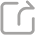分享至QQ空间 分享至朋友圈 分享至新浪微博

### Pullback exponential attractors and a subquintic wave equation Ⅰ

Professor Ma Tofu is from University of Brasilia, works on wave equations.

In this series of lectures, we recall the concept of exponential at- tractors and introduce a recent existence theory (by Carvalho and Sonner) which is specially dedicated to wave type equa- tions. It can be used for either autonomous or non-autonomous systems. Then we apply the theory to some weakly damped Timoshenko like systems. Our main concern is the existence of finite-dimensional dynamics of subquintic wave equations. To this end we present a new proof for the well-posedness of a non- autonomous subquintc wave equation and then apply above theory to show the existence of a pullback exponential attractor. Finally, we also present some recent results and open problems.